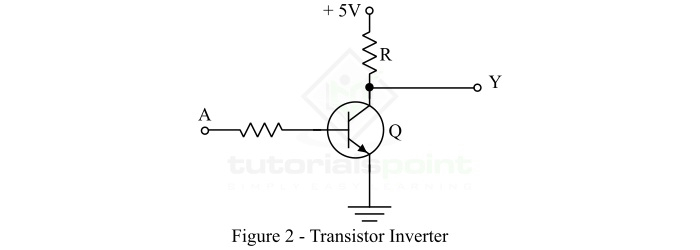# Build an Inverter with a Transistor

In this tutorial, we will discuss the realization of an inverter (NOT gate) with a transistor. But before that let's have a look into the basics of inverter or NOT gate.

## What is an Inverter (NOT Gate)?

An inverter is a single input and single output logic gate that toggles or inverts the logic level (or state) of a single to the opposite state or logic level. The inverter is also known as NOT gate.

When a low signal (logic 0) is applied to an inverter, it transforms it to a high signal (logic 1). If a high signal (logic 1) is applied to the inverter, then it converts it to a low signal (logic 0). Thus, the inverter (NOT gate) alters the logic state of the input signal to its opposite logic state.Therefore, an inverter is basically a device whose output is always the inversion (or complement) of its input. The standard symbol of the inverter is shown in figure-1. The NOT operation is often represented by a bar (-) symbol. Thus, if A is the input variable to the inverter and Y is the output variable of the inverter, then the expression for the output of the inverter is given by,

$$\mathrm{Y=\bar{A}}$$

It is read as Y is equal to A bar.

### Truth Table of NOT Gate (Inverter)

The following is the truth table of the NOT gate (inverter) −

Input (A)

Output (Y = A')

0

1

1

0

We can realize an inverter in many ways like using OR gate, AND gate, NAND gate, NOR gate, etc. An inverter may also be created using a transistor, which will discuss in this article.

## Build an Inverter with a Transistor

As we known, a transistor can function as a switch, i.e., ON switch in its saturation state and OFF switch in its cut off state. Due to this characteristic of the transistor, we may use it as a switch to perform inversion (NOT gate) operation. The circuit diagram of a transistor inverter is shown in Figure-2.Here, we used the transistor in its common emitter configuration. In the common emitter configuration, the transistor provides a phase shift of 180° between input and output. Due to this 180° phase shift, the transistor can produce a high signal (logic 1) at the output when the applied input is low, and it can produce a low signal (logic 0) at the output when the applied input is high. In this way, a transistor work like an inverter and produces inverted value at the output.

### Operation and Truth Table of Transistor Inverter

The input to the transistor inverter may be 0 V or +5 V. Thus, when the input A is at 0 V, the transistor Q is OFF. Therefore, no current flows through the resistor R, and hence there is no voltage drop across it. As a result, the output voltage Y = +5 V. When A = +5 V, the transistor Q is ON and the output voltage Y = 0 V. The truth table for the transistor inverter is shown below.

Input (A)

Output (Y)

0 V

5 V

5 V

0 V

Hence, in this way, we can build an inverter with a transistor. Where, the transistor is used in the common emitter configuration.

Updated on: 10-Jan-2023

2K+ Views